# The PHREG Procedure

### Influence of Observations on Overall Fit of the Model

The LD statistic approximates the likelihood displacement, which is the amount by which minus twice the log likelihood (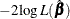), under a fitted model, changes when each subject in turn is left out. When the ith subject is omitted, the likelihood displacement is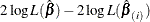where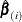is the vector of parameter estimates obtained by fitting the model without the ith subject. Instead of refitting the model without the ith subject, Pettitt and Bin Daud (1989) propose that the likelihood displacement for the ith subject be approximated by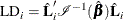where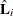is the score residual vector of the ith subject. This approximation is output to the LD= variable.

The LMAX statistic is another global influence statistic. This statistic is based on the symmetric matrix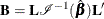whereis the matrix with rows that are the score residual vectors. The elements of the eigenvector associated with the largest eigenvalue of the matrix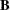, standardized to unit length, give a measure of the sensitivity of the fit of the model to each observation in the data. The influence of the ith subject on the global fit of the model is proportional to the magnitude of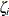, whereis the ith element of the vector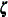that satisfies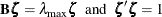with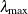being the largest eigenvalue of. The sign ofis irrelevant, and its absolute value is output to the LMAX= variable.

When the counting process MODEL specification is used, the LD= and LMAX= variables are set to missing, because these two global influence statistics can be calculated on a per-subject basis only.